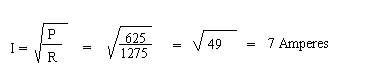## Calculating With Ohm's Law

This guide shows you the proper formula to use to calculate with Ohm's Law.

### A. When Volts and OHMS are known:

 Amperes = VoltsOHMS or, I = ER

Example: Find the current of a 120 volt circuit with a resistance of 60 OHMS.

 I = E R = 120 60 = 2 Amperes

### B. When Watts and Volts are known:

 Amperes = Watts Volts or, I = P E

Example:A 120 Volt Circuit has a 1440 Watt Load. Determine the current.

 I = P E = 1440 120 = 12 Amperes

###Example: A circuit consumes 625 watts through a 12.75 OHM resistor. Determine the current.* One Electrical Horsepower = 746 watts. Electric Motors are rated in horsepower.
** One kilowatt = 1000 watts. Generators are rated in kilowatts.